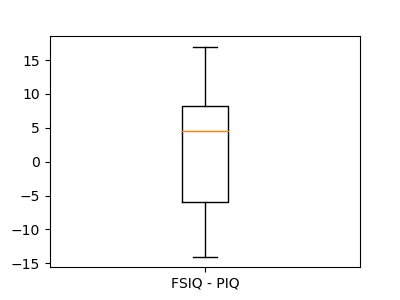# 3.1.6.1. Boxplots and paired differences¶

Plot boxplots for FSIQ, PIQ, and the paired difference between the two: while the spread (error bars) for FSIQ and PIQ are very large, there is a systematic (common) effect due to the subjects. This effect is cancelled out in the difference and the spread of the difference (“paired” by subject) is much smaller than the spread of the individual measures.

••```import pandas

import matplotlib.pyplot as plt

# Box plot of FSIQ and PIQ (different measures od IQ)
plt.figure(figsize=(4, 3))
data.boxplot(column=['FSIQ', 'PIQ'])

# Boxplot of the difference
plt.figure(figsize=(4, 3))
plt.boxplot(data['FSIQ'] - data['PIQ'])
plt.xticks((1, ), ('FSIQ - PIQ', ))

plt.show()
```

Total running time of the script: ( 0 minutes 0.202 seconds)

Gallery generated by Sphinx-Gallery